Courses

# Past Year Questions: Theory of Column & Shear Centre Notes | EduRev

## Civil Engineering (CE) : Past Year Questions: Theory of Column & Shear Centre Notes | EduRev

The document Past Year Questions: Theory of Column & Shear Centre Notes | EduRev is a part of the Civil Engineering (CE) Course Solid Mechanics.
All you need of Civil Engineering (CE) at this link: Civil Engineering (CE)

Q.1 A column of height h with a rectangular cross-section of size a x 2a has a buckling load of P. If the cross-section is changed to 0.5 a x 3a and its height changed to 1.5h, the buckling load of the redesigned column will be    [2018 : 1 Mark, Set-I]
(a) P/12
(b) P/4
(c) P/2
(d) 3P/4
Ans.
(a)
Solution:
For column,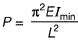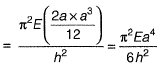For new column,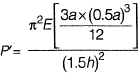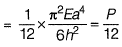Q.2 Consider two axially loaded columns, namely, 1 and 2, made of a linear elastic material with Young’s modulus 2 x 105 MPa, square cross- section with side 10 mm, and length 1 m. For Column 1, one end is fixed and the other end is free. For Column 2, one end is fixed and the other end is pinned. Based on the Euler’s theory, the ratio (up to one decimal place) of the buckling load of Column 2 to the buckling load of Column 1 is _____.    [2017 : 2 Marks, Set-I]
Solution: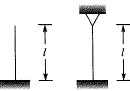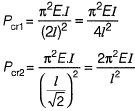∴ Required ratio =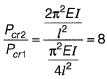Q.3 In a system two connected rigid bars AC and BC are of identical length, L with pin supports at A and B. The bars are interconnected at C by a frictionless hinge. The rotation of the hinge is restrained by a rotational spring of stiffness, k. The system initially assumes a straight line configuration, ACB. Assuming both the bars as weightless, the rotation at supports, A and B, due to a transverse load, P applied at C is    [2015 : 2 Marks, Set-II]
(a) PL/4k
(b) PL/2k
(c) P/4k
(d) Pk/4L
Ans.
(A)
Solution:
Deflection under load P= θL
Work done by force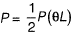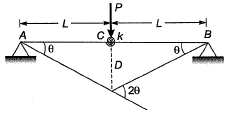Strain energy stored in spring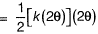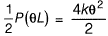⇒ θ = PL/4k

Q.4  If the following equation establishes equilibrium in slightly bent position, the mid-span deflection of a member shown in the figure is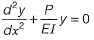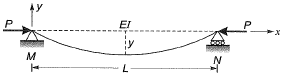If a is amplitude constant for y, then   [2014 : 2 Marks, Set-I]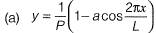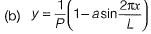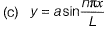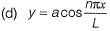Ans.
(C)
Solution: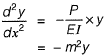∴ Solution of above differential equation is,
y = a sin mx + b cos mx
at = x = 0, y = 0
⇒ b = 0
at x = L, y = 0
⇒ 0 = sin mL
⇒ mL = nπ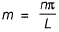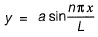Q.5 The possible location of shear centre of the channel section, shown below is,    [2014 : 1 Mark, Set-I]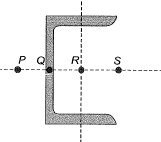(a) P
(b) Q
(c) P
(d) S
Ans.
(A)
Solution: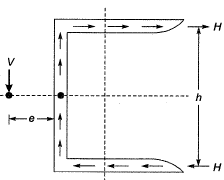For no twisting
V x e = H x h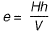Hence possible location of shear centre is P.

Q.6 Two steel column P (length L and yield strength fy = 250 MPa) and Q (length 2L and yield strength fy = 500 MPa) have the same cross-section and end condition the ratio of buckling load of column P to that of column Q is    [2013 : 1 Mark]
(a) 0.5
(b) 1.0
(c) 2.0
(d) 4.0
Ans.
(D)
Solution:
Buckling load,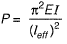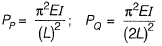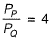Q.7 The ratio of the theoretical critical buckling load for a column with fixed ends to that of another column with the same dimensions and material, but with pinned ends, is equal to    [2012 : 1 Mark]
(a) 0.5
(b) 1.0
(c) 2.0
(d) 4.0
Ans.
(D)
Solution:
Eulars critical load,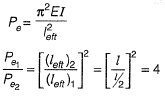Offer running on EduRev: Apply code STAYHOME200 to get INR 200 off on our premium plan EduRev Infinity!

## Solid Mechanics

31 videos|27 docs|29 tests

,

,

,

,

,

,

,

,

,

,

,

,

,

,

,

,

,

,

,

,

,

;